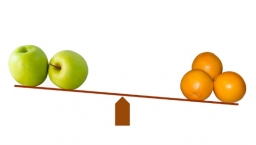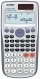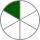# Comparing and sorting

Arrange in descending order this fractions:

2/7, 7/10 & 1/2

Result

a = (Correct answer is: 2/7 < 1/2 < 7/10)### Step-by-step explanation:Did you find an error or inaccuracy? Feel free to write us. Thank you!Tips to related online calculators
Need help to calculate sum, simplify or multiply fractions? Try our fraction calculator.

## Related math problems and questions:

• ArrangeArrange the following in descending order: 0.32, 2on5, 27%, 1 on 3
• Sorting ASCWhich group of decimals is in order from least to greatest? A) 1.04, 0.39, 0.8, 2.1, 0.09 B) 2.1, 1.04, 0.39, 0.8, 0.09 C) 0.09, 0.39, 0.8, 1.04, 2.1 D) 0.09, 0.39, 0.8, 2.1, 1.04
• CompareCompare fractions (34)/(3) and (12)/(4). Which fraction of the lower?
• FractionFind for what x fraction (-4x -6)/(x) equals:
• Simplest form of a fractionWhich one of the following fraction after reducing in simplest form is not equal to 3/2? a) 15/20 b) 12/8 c) 27/18 d) 6/4
• FractionsSort fractions z1 = (6)/(11); z2 = (10)/(21); z3 = (19)/(22) by its size. Result write as three serial numbers 1,2,3.
• Without 2Without multipying, tell whether the product 0.644 x 0.25 will be greater than 1 or less than 1? Explane how you know. Then find the product.
• Sort fractionsWhich is larger 3/7, 3/8, 3/9, 3/6 =
• RegroupingSubtract mixed number with regrouping: 11 17/20- 6 19/20
• Two numbers and its productThe product of two numbers are 2/3. If on of them is 1/10, what is the other?
• Equivalent fractionsAre these two fractions equivalent -4/9 and 11/15?
• Colored blocksTucker and his classmates placed colored blocks on a scale during a science lab. The brown block weighed 8.94 pounds, and the red block weighed 1.87 pounds. How much more did the brown block weigh than the red block?
• One sixthHow many sixths are two thirds?
• Two numbers 11The sum of two rational numbers is (-2). If one of them is 3/5, find the other.
• How manyHow many integers are greater than 547/3 and less than 931/4?
• ExpressionIf it is true that (l + 15 w)/(w) = 6, then the value of the expression (13 l)/(12 w) is:How much and how many times is 72.1 greater than 0.00721?